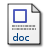# A lamination parameter-based strategy for solving an integer-continuous problem arising in composite optimization

Liu, D. and Toropov, V.V. (2013) A lamination parameter-based strategy for solving an integer-continuous problem arising in composite optimization. Computers and Structures, 128. pp. 170-174. ISSN 0045-7949Microsoft Word (Author version for Computer and Structures Journal) - Accepted Version Download (332kB)

## Abstract

A bi-level optimization strategy for finding the optimal ply numbers and stacking sequence in composite structures has become one of the most popular techniques in recent years. When the optimization technique is based on the use of lamination parameters, the top level optimization has two subsets of design variables for each substructure (e.g., a panel in wing design): lamination parameters treated as continuous design variables, and three integers that define the number of plies of 0, 90 and ±45 orientation. When a continuous optimizer is used at the top level, there is a need for an algorithm to find an integer representation of the obtained continuous number of plies that, ideally, does not alter the mechanical performance of a panel. The focus of this paper is on solving the top level optimization problem whereas the description of local level optimization problem that arranges the stacking sequence can be found in the authors' previous work. In order to determine the integer values of the ply numbers, two schemes based on the lamination parameter matching are introduced in this paper. The strategy is to use a binary code controlling the integer representation of ply numbers in order to obtain a discrete number of plies of each orientation per composite panel. An optimization problem is formulated where the objective function (to be minimized) defines how close the lamination parameter values and the panel thickness, obtained in the top level optimization, are to their values when integer ply numbers are considered. Such an optimization problem is solved by a permutation GA for each individual panel. A wing box benchmark problem is used to demonstrate the potential of these methods.

Item Type: Article optimization,integer representation,lamination parameters,laminated composite Faculty of Science > School of Mathematics http://www.scopus.com/inward/record.url?... Pure Connector 24 Jul 2015 23:03 21 Oct 2022 01:02 https://ueaeprints.uea.ac.uk/id/eprint/53801 10.1016/j.compstruc.2013.06.003View Item